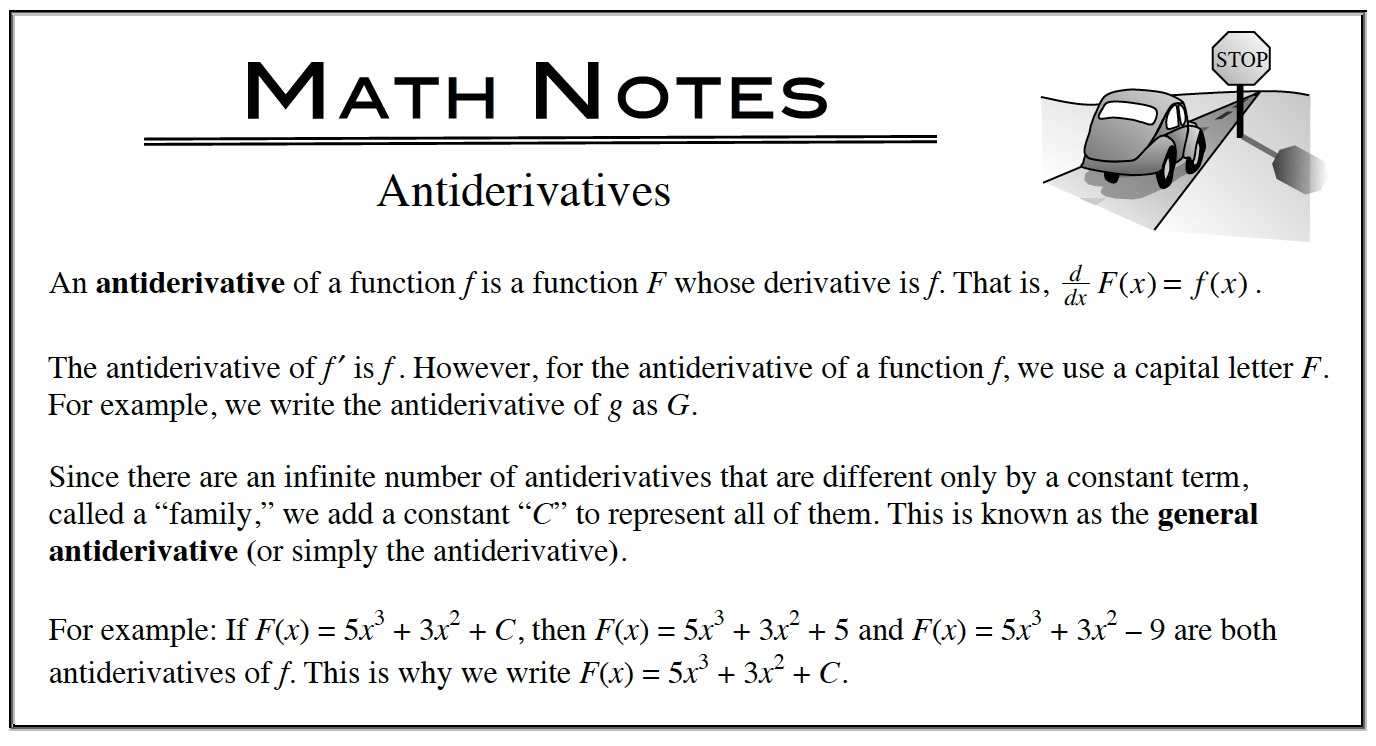Home > APCALC > Chapter 7 > Lesson 7.3.5 > Problem7-154

7-154.

For each of the derivative functions below, write the equations of two different possible functions for $f(x)$. Homework Help ✎

1. $f^\prime(x) = 1$

2. $f^\prime(x) = x^2$